# Friction in Solids

Types of Friction

Static Friction: Friction acting on an object so as to keep it static.

Kinetic Friction: Friction acting on a moving object so as to reduce its velocity. The direction of Kinetic Frictional Force is in opposite direction to its motion.

Rolling Friction: Friction acting on Rolling objects.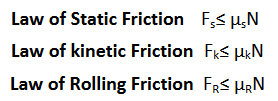Angle of Friction: -

The angle made by the resultant reaction force with the vertical (normal reaction) is known as the angle of friction.

Now, in the triangle OAB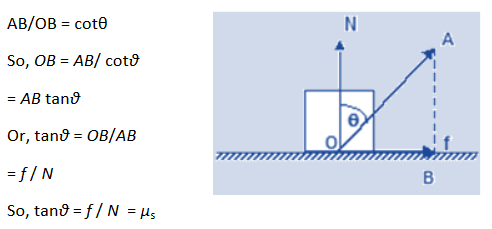Angle of Repose:

It is the angle which an inclined plane makes with the horizontal so that a body placed over it just begins to slide of its own accord.

Consider a body of mass m resting on an inclined plane of inclination q. The forces acting on the body are shown –Ff being the force of friction. If friction is large enough, the body will not slide down.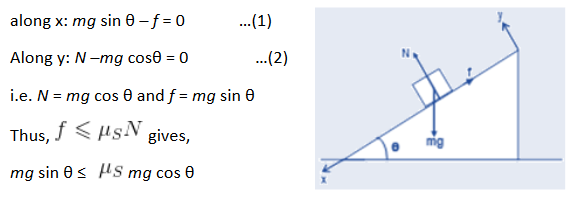So, tanθ ≤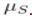This signifies, the coefficient of static friction between the two surfaces, in order that the body doesn’t slide down.

When q is increased, then tan θ >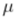.  Thus sliding begins, and the angle θr = tan 1. This angle is known as the angle of repose.

Post By : Ravindra Yadav 03 Nov, 2019 2408 views Physics